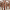# Imaginary replica analysis of loopy regular random graphs

The imaginary replica method provides a new approach for calculating the spectral properties of an ensemble of random regular loopy graphs.

Submitted to Journal of Physics A (2019)

F. Lopez, T. CoolenWe present an analytical approach for describing random loopy regular graphs. By controlling the distribution of eigenvalues of the graph adjacency matrices we control the loopiness of the ensemble. We do this in an effort to bridge the gap between traditional tree like random graph models and real life loopy networks. We derive an expression for the leading two orders of the expected eigenvalue spectrum, through the use of infinitely many replica indices taking imaginary values. We apply the method to models in which the spectral constraint reduces to a soft constraint on the number of triangles, to models with controlled numbers of triangles and squares, and other more complicated ones. Interestingly for practitioners of network science, these models may exhibit a shattering transition where the graphs show an extensive number of disconnected cliques, critically changing its topology.#### Imaginary replica analysis of loopy regular random graphs

F. Lopez, T. Coolen

Sub. to Journal of Physics A

1 / 122 papers